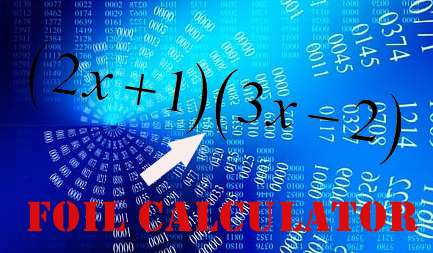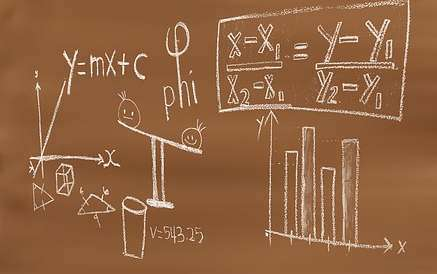# FOIL Calculator

Instructions: Use this calculator to apply FOIL to a suitable expression of the form (a+b)*(c+d), showing all the steps. Please type in the expression you want to carry out a FOIL in the form box below.Enter the expression for which you want to apply FOIL (Ex: (2/3+4/5)*(1/3+3/2), etc.)

This calculator will allow you to conduct FOIL to an expression that requires to be expanded. You need to provide a valid function that requires expansion, of the form (a+b)*(c+d), for example, something like '(1/3+1/4)*(1/5+1/6)'.

Then, we you have a suitable expression typed in, you just have to click on the "Calculate" button to get all the steps of the process shown.

FOILS applies when you want to multiply two binomials, which stands for "F" = first, "O" = Outer, "I" = Inner, "L" = Last, which is a mnemonic to the way the distributive property pans out in the case of two binomials.## How to use the FOIL formula

FOIL is based on the distributive property, and it boils down to the following formula:

$\displaystyle (a+b)(c+d)= ac + ad + bc + bd$

The FOIL process is linked to the idea of using a factor calculator, only that factoring is a type of reverse FOIL.

## What are the steps for FOIL

• Step 1: Make sure you have two binomials that you are multiplying. Otherwise, FOIL does not apply
• Step 2: Identify the binomials as a + b and c + d, so you are computing (a+b)*(c+d)
• Step 3: Using the First, Outer, Inner and Last methodology, use that (a+b)*(c+d) = ac + ad + bc + bd

In other words, the FOIL method is another way of referring to the process of multiplying two binomials.

## Is this a multiplying binomials calculator??

Yes it is, as that is exactly what FOIL does: it creates a methodology to compute the multiplication of binomials. And, the same idea applies if you want to multiply trinomials and so on.

For example, for the simplest case of trinomials, FOIL would indicate that

$\displaystyle (a+b+c)(d+e+f)= ad + ae + af + bd + be + bf + cd + ce + cf$## Is FOIL the same as reverse FOIL

No, though they are tightly related. As the name indicates, one is the reverse of the other. FOIL is about taking to binomials and multiplying them. And reverse FOIL is about factoring an expression to get a multiplication of binomials.

The center of all algebraic calculator starts with the power of basic numbers of fractions.

Observe that FOIL applies for all types of numbers, including imaginary numbers. All sets of numbers with the distributive property are suited for FOIL.### Example: Use FOIL

Apply the FOIL method to compute: $$\left(\frac{1}{3} + \frac{5}{4}\right)\left( \frac{5}{6} + \frac{4}{3} \right)$$

Solution:

We need to apply FOIL to: $$\displaystyle \left(\frac13+\frac54\right)\left(\frac56+\frac43\right)$$. Observe that:

$$\displaystyle \left(\frac{1}{3}+\frac{5}{4}\right)\left(\frac{5}{6}+\frac{4}{3}\right)$$
Note that $$(\frac{1}{3}+\frac{5}{4}) \cdot (\frac{5}{6}+\frac{4}{3}) = \frac{1}{3}\cdot\frac{5}{6}+\frac{1}{3}\cdot\frac{4}{3}+\frac{5}{4}\cdot\frac{5}{6}+\frac{5}{4}\cdot\frac{4}{3} = \frac{247}{72}$$, due to the fact that we can use the distributive property on each term of the expression on the left, with respect to the terms on the right
$$\displaystyle = \,\,$$
$$\displaystyle \frac{247}{72}$$

## More Algebra calculators

FOIL is just a simple methodology to aid students at using the distributive property. You can use this simplify calculator to conduct general expression simplification that include FOIL and many other methods .

Then, the Reverse FOIL is a name for a factoring calculator methodology, in order to group terms together.

The idea of reducing polynomials and calculating roots of polynomials is also a very important one in Algebra.